# Hedging Uniswap V3

Numoen has created first ever, easy to use product for hedging Uniswap LP loss called Numoen Nest. You can read more about it here.
As mentioned in the article, the ability to hedge every single Uniswap LP comes from the innovation of the Power Market Maker Protocol (PMMP). This is a fully decentralized AMM for minting Power Tokens which are option-like derivatives on any token that have Positive Gamma.

## Hedging Process

Hedging the impermanent loss of a Uniswap LP simply entails canceling out the negative gamma of a LP share with the positive gamma of a Power Token.
For the example above we take a ETH/USDC LP position with the following parameters:
ETH Price: \$1825.53
USDC Price:\$1
Lower Bound: \$1600
Upper Bound: \$2000
So to hedge the following Uniswap LP position we:
1. 1.
Calculate the price of a Power Token
Becuase every Power Token (PT) is a quadratic contract, we know that it will square the price of an underlying asset, “squared leverage.” In fact, we also know that the gamma will always be a constant 2 (this useful for the next step).
The price of a PT = (Price of the underlying asset)².
If ETH = \$1825.53, then the price of a PT = (1825.53)² = 3332559.78
2. Calculate the value of a Uniswap LP
With Uniswap V3, the valuing a LP share get a bit more tricky as there is a lower bound and upper bound which refer to the prices between which the liquidity is concentrated on the AMM.
The value of a Uniswap LP is defined as: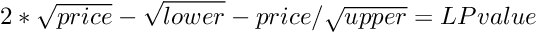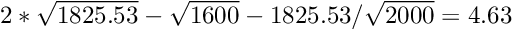3. Calculate the gamma of Uniswap LP
Assuming that the price of the underlying asset is with in the lower and upper bound 0, then the gamma will be: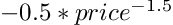If the price of ETH = \$1825.53, then the gamma of the Uniswap LP is: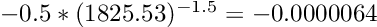4. Calculate the number of Power Tokens.
Using the positive gamma value and price of a Power Token we find the number of Power Tokens needed to hedge a Uniswap LP position.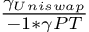Which is the gamma of Uniswap over the negative gamma of a Power Token. Therefore the number of PT needed is: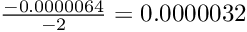## Total Returns

After analyzing the on-chain data for the 7 days `block.timestamp` we can chart the value of the hedged portfolio versus a non-hedge portfolio or “LP Value.” We show that the hedge portfolio [ETH/USDC LP] consistently outperforms across wide and tight bounds.